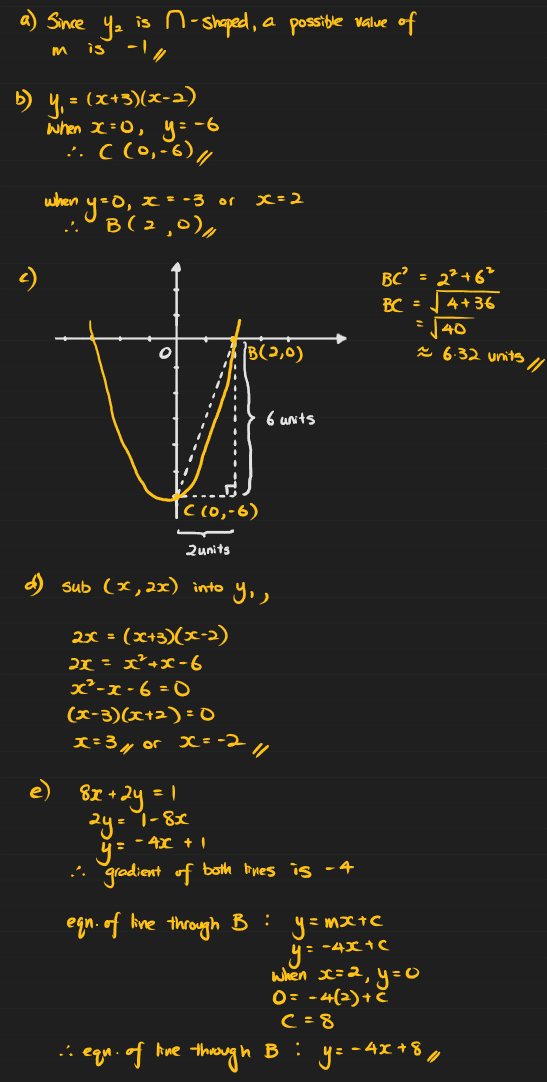# Answer2 Replies 1 Like ✔Accepted Answer

I suppose it could be any negative value. However, I picked -1 since the width of the y2 parabola seems to be about the same as the y1 parabola which has an x2 coefficient of +1. Any other value would make the parabola have different widths.

Also realised I forgot to give a possible value of k. Since the y2 parabola is above the x-axis, it means it has been moved up a few units. So k could be any positive value.

So for me, a possible value set of m and k could be -1 and 2 respectively.

0 Replies 1 Like